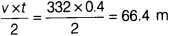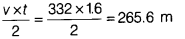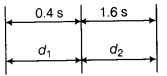# Calculate the distance between buildings

A man stands between two high rise buildings and blows a whistle. He hears two successive echoes after 0.4 s and 1.6 s. Calculate the distance between buildings. [Take, speed of sound = 332 ms–1]

Distance of person from nearer building, d1 =Distance of person from farther building, d2 =Total distance between buildings. = d1 + d2 = 66.4 + 265.6 = 332 m